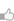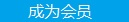求知 文章 文库 Lib 视频 iProcess 课程 角色 咨询 工具 讲座 Modeler Code要资料追随技术信仰 随时听讲座 每天看新闻

 Tensorflow教程 1. 新手入门 1. 1 介绍 1. 2 下载与安装 1. 3 基本用法 2. 完整教程 2. 1 总览 2. 2 MNIST 数据下载 2. 3 MNIST机器学习入门 2. 4 深入MNIST 2. 5 TensorFlow运作方式入门 2. 6 卷积神经网络 2. 7 字词的向量表示 2. 8 递归神经网络 2. 9 曼德布洛特集合 2. 10 偏微分方程 3 进阶指南 3. 1总览 3. 2变量:创建、初始化、保存和加载 3. 3TensorBoard:可视化学习 3. 4TensorBoard: 图表可视化 3. 5数据读取 3. 6线程和队列 3. 7增加一个新 Op 3. 8自定义数据读取 3. 9使用 GPUs 3. 10共享变量 4.资源 4.1总览 4.2BibTex 引用 4.3示例使用 4.4FAQ 4.5术语表 4.6 TENSOR排名、形状和类型

 202 次浏览16次捐助

 def my_image_filter(input_images): conv1_weights = tf.Variable(tf.random_normal([5, 5, 32, 32]), name="conv1_weights") conv1_biases = tf.Variable(tf.zeros(), name="conv1_biases") conv1 = tf.nn.conv2d(input_images, conv1_weights, strides=[1, 1, 1, 1], padding='SAME') relu1 = tf.nn.relu(conv1 + conv1_biases) conv2_weights = tf.Variable(tf.random_normal([5, 5, 32, 32]), name="conv2_weights") conv2_biases = tf.Variable(tf.zeros(), name="conv2_biases") conv2 = tf.nn.conv2d(relu1, conv2_weights, strides=[1, 1, 1, 1], padding='SAME') return tf.nn.relu(conv2 + conv2_biases)

 # First call creates one set of variables. result1 = my_image_filter(image1) # Another set is created in the second call. result2 = my_image_filter(image2)

 variables_dict = { "conv1_weights": tf.Variable(tf.random_normal([5, 5, 32, 32]), name="conv1_weights") "conv1_biases": tf.Variable(tf.zeros(), name="conv1_biases") ... etc. ... } def my_image_filter(input_images, variables_dict): conv1 = tf.nn.conv2d(input_images, variables_dict["conv1_weights"], strides=[1, 1, 1, 1], padding='SAME') relu1 = tf.nn.relu(conv1 + variables_dict["conv1_biases"]) conv2 = tf.nn.conv2d(relu1, variables_dict["conv2_weights"], strides=[1, 1, 1, 1], padding='SAME') return tf.nn.relu(conv2 + variables_dict["conv2_biases"]) # The 2 calls to my_image_filter() now use the same variables result1 = my_image_filter(image1, variables_dict) result2 = my_image_filter(image2, variables_dict)

tf.get_variable(<name>, <shape>, <initializer>): 通过所给的名字创建或是返回一个变量.

tf.variable_scope(<scope_name>): 通过 tf.get_variable()为变量名指定命名空间.

tf.constant_initializer(value) 初始化一切所提供的值,

tf.random_uniform_initializer(a, b)从a到b均匀初始化,

tf.random_normal_initializer(mean, stddev) 用所给平均值和标准差初始化均匀分布.

 def conv_relu(input, kernel_shape, bias_shape): # Create variable named "weights". weights = tf.get_variable("weights", kernel_shape, initializer=tf.random_normal_initializer()) # Create variable named "biases". biases = tf.get_variable("biases", bias_shape, initializer=tf.constant_intializer(0.0)) conv = tf.nn.conv2d(input, weights, strides=[1, 1, 1, 1], padding='SAME') return tf.nn.relu(conv + biases)

 def my_image_filter(input_images): with tf.variable_scope("conv1"): # Variables created here will be named "conv1/weights", "conv1/biases". relu1 = conv_relu(input_images, [5, 5, 32, 32], ) with tf.variable_scope("conv2"): # Variables created here will be named "conv2/weights", "conv2/biases". return conv_relu(relu1, [5, 5, 32, 32], )

 result1 = my_image_filter(image1) result2 = my_image_filter(image2) # Raises ValueError(... conv1/weights already exists ...)

 with tf.variable_scope("image_filters") as scope: result1 = my_image_filter(image1) scope.reuse_variables() result2 = my_image_filter(image2)

 v = tf.get_variable(name, shape, dtype, initializer)

 with tf.variable_scope("foo"): v = tf.get_variable("v", ) assert v.name == "foo/v:0"

 with tf.variable_scope("foo"): v = tf.get_variable("v", ) with tf.variable_scope("foo", reuse=True): v1 = tf.get_variable("v", ) assert v1 == v

tf.variable_scope() 基础

 with tf.variable_scope("foo"): with tf.variable_scope("bar"): v = tf.get_variable("v", ) assert v.name == "foo/bar/v:0"

 with tf.variable_scope("foo"): v = tf.get_variable("v", ) tf.get_variable_scope().reuse_variables() v1 = tf.get_variable("v", ) assert v1 == v

 with tf.variable_scope("root"): # At start, the scope is not reusing. assert tf.get_variable_scope().reuse == False with tf.variable_scope("foo"): # Opened a sub-scope, still not reusing. assert tf.get_variable_scope().reuse == False with tf.variable_scope("foo", reuse=True): # Explicitly opened a reusing scope. assert tf.get_variable_scope().reuse == True with tf.variable_scope("bar"): # Now sub-scope inherits the reuse flag. assert tf.get_variable_scope().reuse == True # Exited the reusing scope, back to a non-reusing one. assert tf.get_variable_scope().reuse == False

 with tf.variable_scope("foo") as foo_scope: v = tf.get_variable("v", ) with tf.variable_scope(foo_scope) w = tf.get_variable("w", ) with tf.variable_scope(foo_scope, reuse=True) v1 = tf.get_variable("v", ) w1 = tf.get_variable("w", ) assert v1 == v assert w1 == w

 with tf.variable_scope("foo") as foo_scope: assert foo_scope.name == "foo" with tf.variable_scope("bar") with tf.variable_scope("baz") as other_scope: assert other_scope.name == "bar/baz" with tf.variable_scope(foo_scope) as foo_scope2: assert foo_scope2.name == "foo" # Not changed.

 with tf.variable_scope("foo", initializer=tf.constant_initializer(0.4)): v = tf.get_variable("v", ) assert v.eval() == 0.4 # Default initializer as set above. w = tf.get_variable("w", , initializer=tf.constant_initializer(0.3)): assert w.eval() == 0.3 # Specific initializer overrides the default. with tf.variable_scope("bar"): v = tf.get_variable("v", ) assert v.eval() == 0.4 # Inherited default initializer. with tf.variable_scope("baz", initializer=tf.constant_initializer(0.2)): v = tf.get_variable("v", ) assert v.eval() == 0.2 # Changed default initializer.

 with tf.variable_scope("foo"): x = 1.0 + tf.get_variable("v", ) assert x.op.name == "foo/add"

 with tf.variable_scope("foo"): with tf.name_scope("bar"): v = tf.get_variable("v", ) x = 1.0 + v assert v.name == "foo/v:0" assert x.op.name == "foo/bar/add"

 File What's in it? models/image/cifar10.py 图像中检测对象的模型. models/rnn/rnn_cell.py 时间递归神经网络的元方法集. models/rnn/seq2seq.py 为创建sequence-to-sequence模型的方法集.
 您可以捐助，支持我们的公益事业。 1元 10元 50元 认证码：必填
 202 次浏览16次捐助每天2个文档/视频 扫描微信二维码订阅
 订阅技术月刊 获得每月300个技术资源